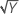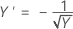# Methods for Stability Study for random batches

Select the method of your choice.

## The mixed model and log-likelihood

### The general form of the mixed model

Mixed effect models contain both fixed and random effects. The general form of the mixed effect model is:

y =+ Z1μ1 + Z2μ2 + ... + Zcμc + ε

### Notation

TermDescription
ythe n x 1 vector of response values
Xthe n x p design matrix for the fixed effects, pn
Zithe n x mi design matrix for the ith random effect in the model
βa p x 1 vector of unknown parameters
μian mi x 1 vector of independent variables from N(0, σ2i)
εan n x 1 vector of independent variables from N(0, σ2i)
cthe number of random effects in the model

### Particular forms of the mixed model

Stability studies fits two models with a random batch factor. The largest model contains time, the random batch factor, and the random interaction between time and batch.

y =+ Z1μ1 + Z2μ2 + ε

The smaller model contains time and the random batch factor.

y =+ Z1μ1 + ε

The general variance-covariance matrix of the response vector, y, is:

V(σ2) = V(σ2, σ21, ... , σ2c) = σ2In + σ21Z1Z'1 + ... + σ2cZcZ'c

where

σ2 = (σ2, σ21, ... , σ2c)'

σ2, σ21, ... , σ2c are called variance components.

By factoring from the variance, you can find a representation of H(θ), which is in the computation of the log-likelihood of mixed models.

V(σ2) = σ2H(θ) = σ2[In + θ1Z1Z'1 + ... + θcZcZ'c]

When batch is a random factor, the unknown parameter estimates come from minimizing twice the negative of the restricted log-likelihood function. The minimization is equivalent to maximizing the restricted log-likelihood function. The function to minimize is:

### Notation

TermDescription
nthe number of observations
pthe number of parameters in β, 2 for stability studies
σ2the error variance component
Xthe design matrix ––for the fixed terms, the constant and time
H(θ)In + θ1Z1Z'1 + ... + θcZcZ'c
Inthe identity matrix with n rows and columns
θithe ratio of the variance of the ith random term over the error variance
Zithe n x mi matrix of known codings for the ith random effect in the model
mithe number of levels for the ith random effect
cthe number of random effects in the model
|H(θ)|the determinant of H(θ)
X'the transpose of X
H-1(θ)the inverse of H(θ)

## Box-Cox transformation

Box-Cox transformation selects lambda values, as shown below, which minimize the residual sum of squares. The resulting transformation is Y λ when λ ≠ 0 and ln(Y) when λ = 0. When λ < 0, Minitab also multiplies the transformed response by −1 to maintain the order from the untransformed response.

Minitab searches for an optimal value between −2 and 2. Values that fall outside of this interval might not result in a better fit.

Here are some common transformations where Y′ is the transform of the data Y:

Lambda (λ) value Transformation
λ = 2 Y′ = Y 2
λ = 0.5 Y′ =λ = 0 Y′ = ln(Y )
λ = −0.5λ = −1 Y′ = −1 / Y

## Random batch model selection

The model selection determines whether the shelf life depends on batch and whether the effect of time depends on the batch. Minitab considers the following three models in sequence:
1. Time + Batch + Batch*Time (unequal slopes and intercepts for batches)
2. Time + Batch (equal slopes and unequal intercepts for batches)
3. Time (equal slopes and intercepts for batches)

If the Batch*Time interaction is significant, the analysis fits the first model. If the interaction is not significant but the Batch term is significant in the second model, the analysis fits the second model. Otherwise, the analysis fits the third model.

The test for whether to pool batches is slightly different from the test to include batch, although both depend on the chi-square distribution. The formulas for the test statistics and p-values are as follow.

### Test between model 1 and model 2

difference = −2L2 − (−2L1)

p = 0.5 * Prob(χ21 > difference) + 0.5 * Prob(χ22 > difference)

### Test between model 2 and model 3

difference = −2L3 − (−2L2)

p = 0.5 * Prob(χ21 > difference)

### Notation

TermDescription
Lathe log-likelihood for model a
pthe p-value for the test
Prob(χ21> difference)the probability that a random variable from a chi-square distribution with 1 degree of freedom is greater than the difference
Prob(χ22> difference)the probability that a random variable from a chi-square distribution with 2 degrees of freedom is greater than the difference

### References

1. Searle, S.R. Casella, G. and McCuloch, C.E. (1992). Variance Components
2. West, B.T., Welch, K.B. and Galecki, A.T. (2007). Linear Mixed Models: A Practical Guide Using Statistical Software.
3. Chow, S. (2007). Statistical Design and Analysis of Stability Studies.
By using this site you agree to the use of cookies for analytics and personalized content.  Read our policy